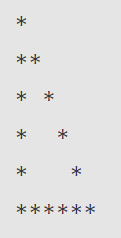Related Tags

javascript
nested for loops
triangle pattern
communitycreator

# How to make a hollow triangle pattern in JavaScript

Ayush Trivedi

In this shot, we will learn how to make a hollow triangle pattern in JavaScript.

### Desired output

We will be printing the pattern shown below:### Code

// total number of rows
let n = 6;

for (let i = 1; i <= n; i++) {
// printing stars and spaces
for (let j = 0; j < i; j++) {
if(i === n) {
process.stdout.write('*')
}
else {
if (j == 0 || j == i - 1) {
process.stdout.write('*')
}
else {
process.stdout.write(' ')
}
}
}
console.log();
}

### Explanation

• Line 1: We initialize a variable n with an integer, which represents the total number of rows that make up the hollow triangle. You can also think of this as the height of the triangle.

• Line 3: We have our first for loop, which will iterate over the total number of rows. In the example above, the number of rows is six.

• Line 5: We have our nested for loop, which will iterate for less than the current row number. For example, when i = 3 in our outer for loop, that means we’re on row three of the triangle. Hence, our nested for loop will run only three times for the following values of j:

• j = 0

• j = 1

• j = 2

• Lines 6 to 8: The condition if(i===n) is used to check if i (current row) is equal to n (last row). This will only be true on the last iteration of the outer for loop. When this condition is true, the entire row is printed, consisting of only *'s and no spaces.

• Line 9: If i is not equal to n, we’re not on the last row of the triangle. Therefore, the else block will execute.

• Lines 10 to 12: Inside the else block, we again have a condition if (j == 0 || j == i - 1) to check if the value of j in the nested for loop is equal to 0 or i-1. If this condition is true, that means we’re at an end point of the current row of the triangle. In this case, we’ll print a * for the row.

• Lines 13 to 15: If the if condition explained directly above is false, then j is either greater than 0 or less than i - 1. This means that we’re currently on an iteration of the nested for loop that is not at either of the endpoints of the current row being traversed. In this case, we’ll execute the nested else block, and print a ' '(space) for the row.

• Line 18: We used console.log() with null, as it will change to a new line. We can also use process.stdout.write('\n') to change the line.

### Conclusion

We successfully completed the task to print the hollow triangle using *'s. However, this is only one way to go about doing this. There can be several algorithms to achieve the same result.

RELATED TAGS

javascript
nested for loops
triangle pattern
communitycreator

CONTRIBUTOR

Ayush Trivedi
RELATED COURSES

View all Courses

Keep Exploring

Learn in-demand tech skills in half the time